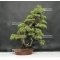##### The Japanese Bonsai specialist
Direct order Contact Help / Services Newsletter# Pinus pentaphylla 20060181

Pinus pentaphyllaref. : 9947

1.630,00

voluminous/heavy item extra shipping of 12,00

Available quantity : 1Order

###### Description

Height of the tree without its pot : 780 mm. Width of the branches : 600mm. Artisanal quality non-enamelled pottery from Yixing of : Ø 415 x 97 mm. Sokan style with two trunks and shari miki. Pine with a regular silhouette, a large trunk perfectly conical and a proportional thinner one, without any strong wire marks or cutting wounds. The trunk’s diameter amounts : 80 mm and its nebari 220 mm.Pinus species from seeding, selected in nature (Yamadori) more than 30 years ago. It is composed of a formed bark because this subject is aged of more than 40-45 years old. Look the beauty of this trunk with a shari miki wood-style and its jins base. Currently ligatured with copper wire.

These pictures were taken in june 2018.

#with 4.4 #trunk 3.5 #pinus 3.5 #pentaphylla 3.1 #yamadoris 2.9 #japanese 2.8 #without 2.7 #bonsai 2.6 #years 2.5 #style 2.5

Formule
(( ROUND((CHAR_LENGTH(b.article_nom)-CHAR_LENGTH(REPLACE(b.article_nom, 'with', '')))/LENGTH('with')) + ROUND((CHAR_LENGTH(b.article_description)-CHAR_LENGTH(REPLACE(b.article_description, 'with', '')))/LENGTH('with')) ) * 4.4) + (( ROUND((CHAR_LENGTH(b.article_nom)-CHAR_LENGTH(REPLACE(b.article_nom, 'pinus', '')))/LENGTH('pinus')) + ROUND((CHAR_LENGTH(b.article_description)-CHAR_LENGTH(REPLACE(b.article_description, 'pinus', '')))/LENGTH('pinus')) ) * 3.5) + (( ROUND((CHAR_LENGTH(b.article_nom)-CHAR_LENGTH(REPLACE(b.article_nom, 'trunk', '')))/LENGTH('trunk')) + ROUND((CHAR_LENGTH(b.article_description)-CHAR_LENGTH(REPLACE(b.article_description, 'trunk', '')))/LENGTH('trunk')) ) * 3.5) + (( ROUND((CHAR_LENGTH(b.article_nom)-CHAR_LENGTH(REPLACE(b.article_nom, 'pentaphylla', '')))/LENGTH('pentaphylla')) + ROUND((CHAR_LENGTH(b.article_description)-CHAR_LENGTH(REPLACE(b.article_description, 'pentaphylla', '')))/LENGTH('pentaphylla')) ) * 3.1) + (( ROUND((CHAR_LENGTH(b.article_nom)-CHAR_LENGTH(REPLACE(b.article_nom, 'without', '')))/LENGTH('without')) + ROUND((CHAR_LENGTH(b.article_description)-CHAR_LENGTH(REPLACE(b.article_description, 'without', '')))/LENGTH('without')) ) * 2.7) + (( ROUND((CHAR_LENGTH(b.article_nom)-CHAR_LENGTH(REPLACE(b.article_nom, 'shari', '')))/LENGTH('shari')) + ROUND((CHAR_LENGTH(b.article_description)-CHAR_LENGTH(REPLACE(b.article_description, 'shari', '')))/LENGTH('shari')) ) * 2.5) + (( ROUND((CHAR_LENGTH(b.article_nom)-CHAR_LENGTH(REPLACE(b.article_nom, 'years', '')))/LENGTH('years')) + ROUND((CHAR_LENGTH(b.article_description)-CHAR_LENGTH(REPLACE(b.article_description, 'years', '')))/LENGTH('years')) ) * 2.5) + (( ROUND((CHAR_LENGTH(b.article_nom)-CHAR_LENGTH(REPLACE(b.article_nom, 'style', '')))/LENGTH('style')) + ROUND((CHAR_LENGTH(b.article_description)-CHAR_LENGTH(REPLACE(b.article_description, 'style', '')))/LENGTH('style')) ) * 2.5) + (( ROUND((CHAR_LENGTH(b.article_nom)-CHAR_LENGTH(REPLACE(b.article_nom, 'more', '')))/LENGTH('more')) + ROUND((CHAR_LENGTH(b.article_description)-CHAR_LENGTH(REPLACE(b.article_description, 'more', '')))/LENGTH('more')) ) * 2.4) + (( ROUND((CHAR_LENGTH(b.article_nom)-CHAR_LENGTH(REPLACE(b.article_nom, 'miki', '')))/LENGTH('miki')) + ROUND((CHAR_LENGTH(b.article_description)-CHAR_LENGTH(REPLACE(b.article_description, 'miki', '')))/LENGTH('miki')) ) * 2.4)

## Secure payment## Delivery

Our logistic partners :